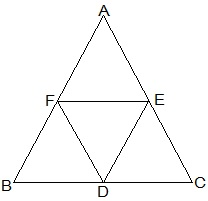Guru

# D, E and F are respectively the mid-points of the sides BC, CA and AB of a ΔABC. Show that (i) BDEF is a parallelogram. Q.5(1)

• 0

Give me the best way for solving the question of class 9th of Areas of Parallelograms and Triangles of exercise 9.3 of math. Give me the easiest way for solving this question in simple way of question no.5 (1) D, E and F are respectively the mid-points of the sides BC, CA and AB of a ΔABC. Show that (i) BDEF is a parallelogram.

Share

1.(i) In ΔABC,

EF || BC and EF = ½ BC (by mid point theorem)

also,

BD = ½ BC (D is the mid point)

So, BD = EF

also,

BF and DE are parallel and equal to each other.

∴, the pair opposite sides are equal in length and parallel to each other.

∴ BDEF is a parallelogram.

• 0